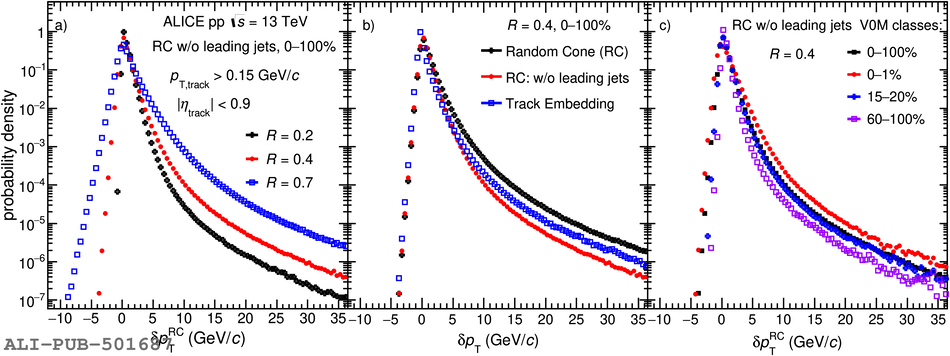# Figure 2

 a) Comparison of the $\delta p_{\rm T}$ distribution obtained for different random cone radii ($R=0.2, 0.4, 0.7$). b) Comparison of the $\delta p_{\rm T}$ distribution with the RC method (including and excluding two leading jets) and the track embedding approach for $R=0.4$. c) Comparison of measured $\delta p_{\rm T}$ distribution using RC method without leading jets for $R=0.4$ in different multiplicity classes .